### Home > PC > Chapter 9 > Lesson 9.2.1 > Problem9-68

9-68.
1. Solve for x in the following equations. Homework Help ✎

1. log3 81 = x

2. 5x =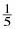3. 3x = 7

4. 92x = 27x−2

5. log2 [log9 (log3 x)] = −1

6. (x − 3)(ln x − 2) = 0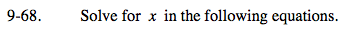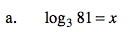3x = 81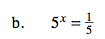5x = 5−1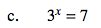log3x = log7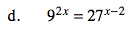(32)2x = (33)x−2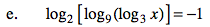2−1 = log9(log3x)

$\text{log}_{9}(\text{log}_{3}x)=\frac{1}{2}$

$9^{1/2}=\log_3x$

$\text{log}_{3}x = 3$

33 = x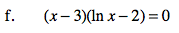x − 3 = 0
Solve for x.

lnx − 2 = 0
Solve for x.